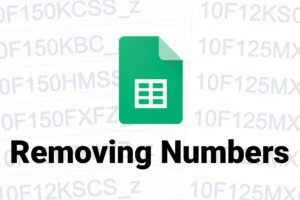# Split & Trim with Google Sheets using ArrayFormula

The issue is white space.

``=split(CELL,"DELIMITER")``

Using the standard SPLIT formula, to get an unwanted white space at the beginning of each cell. Now, I don’t know about you, but that drives me absolutely nuts, and I’m always forgetting the fix. Ultimately, running through this sequence… over and over and over…

In the following example, I’m attempting to use a formula to split the Category column (K), into its subcategories.

Using the standard SPLIT formula you can see, I’m getting an extra white space at the beginning of each cell in columns D, E & F.

``=split(K8,"||")``

It seems that adding an extra space to the end of the delimiter would fix this, only that splits it at every space. I still try to do this sometimes, even though it always fails.

``=split(K8,"|| ")``

Using TRIM with the formula only returns the data up to the first SPLIT, though I frequently repeat this mistake.

``=trim(split(K8,"||"))``

We’re so close here, we’re just missing the ARRAYFORMULA function. (ArrayFormula) “Enables the display of values returned from an array formula into multiple rows and/or columns and the use of non-array functions with arrays.” So, in this case, it basically allows you to apply the TRIM function to each cell populated by your SPLIT.

``=ArrayFormula(trim(split(K8,"||")))``

### Related posts

##### Why isn’t ISNUMBER working in sheets?##### Removing HTML from a Cell in Google Sheets